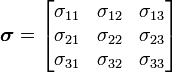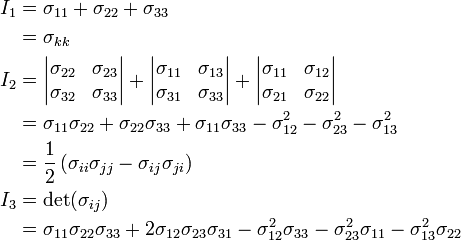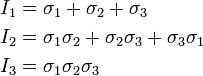# How to calculate 3D Principal stresses?

How to calculate 3D Principal stresses???

If I got the values of 6 stress tensors:Then I can calculate the values for the 3 stress invariants I1, I2 and I3:I also know the relationship between the stress invariants and principal stresses:So how can I calculate the values for the 3 principal stresses?

I can find some simple stress calculator on-line to get the results straight away, but I need the algorithm step for the calculation. In the other word, I need the individual equations for S1, S2 and S3, then I can do the calculation myself without using any software calculator or speardsheet!

Thanks a lot!

## Answers and Replies

Mapes
Science Advisor
Homework Helper
Gold Member
Last edited by a moderator: